#### Number of problems found: 502

• Baking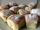There are 28 bunches, and son ate 1/2, dad ate four bunches. How many of them remain on the baking dishes?
• Wire cut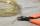A wire of length 7 m was cut into equal lengths using 4 cuts. How long is each piece?
• Garden plotCalculate how many meters of fence need to fence the square garden with length and width of 22 meters.
• BenchesThe park has 64 benches. Occupied are by 18 more than empty. How many benches are occupied and empty ?
• Equation with one variableSolve the following equation with one unknown: 5(7s + 5) =130
• HectaresThe rectangular land is 350m long and 200m wide. Calculate the area in hectares.
• Friends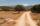Five mates traveled and the journey they lasted 195 minutes. How long would travel only one of them?
• Sum of the digitsHow many are two-digit natural numbers that have the sum of the digits 9?
• One kilogramThe apple weighs 125 grams and half apple. How many apples weigh 1 kilogram?
• Glasses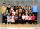Imagine a set of students in your class (number of students: 19), who wears glasses. How much minimum and maximum element may contain this set.
• With bracket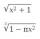Calculate (evaluate) simple mathematical expression with a negative numbers and a bracket: 13+15*5-2*(-6)
• Mom and daughter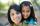Mom is 30 years older than the daughter. What is the age difference between them in 35 years?
• On the farm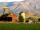The farm took 221 tons of potatoes from 17 hectares. How many tons of potatoes taken from one hectare?
• Cyclist 12What is the average speed of a cycle traveling at 20 km in 60 minutes in km/h?
• Speed of carIn 2 hours 40 mins, a car travels 100km. At what speed is the car traveling?
• Convalescent homes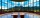In 270 convalescent homes, 94,270 vacationers spent part of the holiday a year. On average, how many vacationers per convalescent home?
• The swallow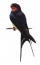The swallow will fly 2.8 km per minute. How many km will the swallow fly in one hour?
• Brother and sisterWhen I was 8, my sister was 4, and now I am 18. How old is my sister?
• MorningJana rose in the morning in the quarter to seven. She slept nine and a half hours. When did she go to sleep?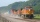The whole loaded train weighs 360 tons. It has twenty wagons, each carrying 12 tons of grain. What is the weight of the locomotive?